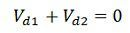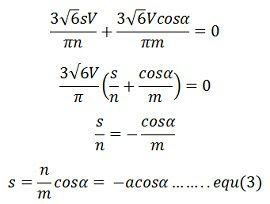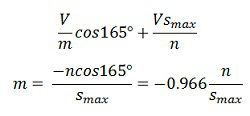# Static Scherbius Drive

The Static Scherbius Drive provides the speed control of a wound rotor motor below synchronous speed. The portion of rotor AC power is converted into DC by a diode bridge. The controlled rectifier works as an inverter and converts the DC power back into AC and feeds it back to the AC source. This drive has the ability of flow the power both in the positive as well as the negative direction of the injected voltage. This increases the operating condition of the drive.

The feedback power is controlled by controlling the inverter counter emf Vd2, which is controlled by controlling the inverter firing angle.The DC link inverter reduced the ripple in DC link current Id. The slip power of the drive is fed back to the source due to which the efficiency of the drive increases.The drive input power is the difference of the DC input power and the power fed back. Reactive input power is the sum of the motor and input reactive power.Thus, the drive has poor power factor throughout the range of its operation.Where α is the inverter firing angle and n, and m are respectively the stator to the rotor turn ratio of motor and source side to convert side turns ratio of the transformer. The neglecting drop across the inductor.Substituting the equation (1) and (2) in the above equation we getwhere a = n/m

The maximum value of alpha is restricted to 165º for safe commutation of inverter thyristor. The slip can be controlled from 0 to 0.966α when α is changed from 90º to 165º.The appropriate speed range can be obtained by choosing the appropriate value of α.

The transformer is used to match the voltage from Vd1 and Vd2. At the lowest speed required from the drive, Vd1 will have the maximum value Vd1m, and it is given byWhere Sm is the value of slip at the lowest speed. If α is restricted to 165, m is chosen such that the inverter voltage has a value Vd1m when α is 165º, i.e.,The value of m determines the highest firing angle at the lower motor speed. It also gives the highest firing angle and the lowest reactive power at the lowest speed.

Considered the circuit of the motor, which is neglecting the magnetising branch. When referred to DC link, resistance (sRs + Rr) will be 2(sR’s + Rr). This gives the equivalent circuit of the drive, where Vd1 and Vd2 are given. Rd is the resistance of the DC link inductor.If rotor copper loss is neglectedThe nature of the speed torque curve is shown in the figure below.The drive has application in pump drive which requires the speed control in the narrow range only. The drive is widely used in medium and high power fan and pump drives, because of high efficiency and low cost.

### Operating Modes of Static Scherbius Drives

The following are the operating modes of Static Scherbius Drives.

Sub-synchronous Motoring – In this mode of operation the slip and torque both are positive and hence the injected voltage is in phase with rotor current. The power flows into the stator and feedback into the rotor circuit.

Super-synchronous Motoring – When the speed of the motor is above the synchronous speed, then the slip is negative. Thus, the voltage and current are out of phase with each other.The power feeds into the rotor from the drive circuit along with input power flowing into the stator.

Sub-synchronous Generating – For sub-synchronous speed, the torque is required to be positive, although the slip is positive. The power is fed into the rotor through the slip ring.

Super-synchronous Generating – When the speed of the motor above the synchronous speed, then the slip and torque becomes negative. Thus, the injecting voltage is in phase with the rotor. The mechanical power is injected by the shaft and the output power is obtained from the stator and rotor circuit.